Share

# Maths Revision GCSE: Quotient Equations

By Yann, published on 20/11/2018 > > > GCSE Maths Revsion: Quotient Equations

An area that trips a lot of students up during their GCSE maths course is quotient equations: this can more easily be expressed as just “an equation with a division operation in it”.

Here’s something you need to remember:

A quotient (ie: a fraction) is zero only if the numerator (top half of the fraction) is zero, and the denominator (bottom half of the fraction) is not zero.

Why is this? Because you can’t divide by zero, and that’s all a fraction (or “quotient”) really is: a way of expressing a division operation!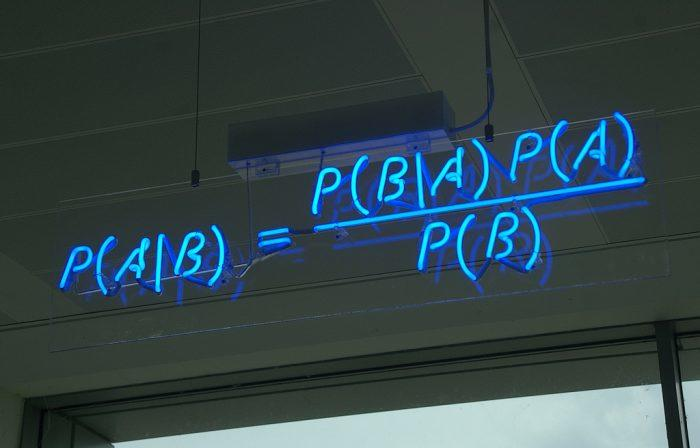Quotients and Fractions will be a doddle after you’ve read our GCSE maths guide. (Photo credit: mattbuck4950 via Visualhunt.com / CC BY-SA)

So now we know that, we can work out the best way to solve the maths problem. And the best way to solve the problem is to get rid of the fraction altogether, as this avoids tripping ourselves up by dividing by zero later.

As you might be able to tell, we’re going to do this using the same method as before. Specifically carrying out operations on both sides of the equation.

So, here’s an example to put all of this into context: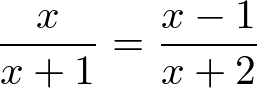Now this looks pretty scary, but it’s not too bad. We have all rational numbers (a fancy way of saying no square roots), and we can follow the same steps as before. Namely: we need to isolate ‘x’, and set the equation equal to zero!

As I said before, we don’t want to divide anything by zero, so let’s get rid of the fractions all together. A fraction is just a way of dividing something, and the opposite of dividing something is multiplying by it.

Let’s start by getting rid of the fraction on the left hand side: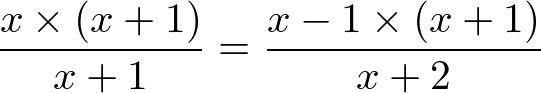Just like in our linear equation example, I multiplied both sides by (x+1). This means that on the left hand side, I now multiply by (x+1) and then immediately divide by (x+1). And just like in our previous example, that means they cancel each other out, and I can remove them from the equation altogether: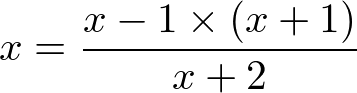So, it’s starting to look a lot nicer already: I’ve now got a single ‘x’ on one side, removed one fraction, and we’re well on our way to solving this problem. Let’s also get rid of the fraction on the right hand side, using the same method:Now, this might look like a step backwards, as it looks a lot uglier than it did before. However, if you look at the right hand side, I’m multiplying and then dividing by (x+2), which, just like before, we can cancel out completely.

Another way we’ll tidy this up is by removing our multiplication symbols. This might seem strange if you’ve not had much experience with equations before – but in an equation, if two things are written directly next to each other, with no sign between them, they’re multiplied.

The following two equations mean exactly the same thing, and from this point on, we’ll be using the format without multiplication signs: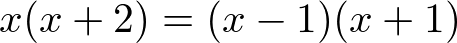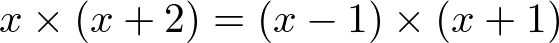It’s looking a lot nicer now, but there’s a lot of x’s in that equation! In fact, if you look closely, you’ll see we’re multiplying x’s together, which will make powers of x.

To continue solving this problem, we’re going to need to take a quick intermission and learn something new!

## Polynomial Equations

There are other types of equation, of course. One of these is polynomial equations (meaning there are powers), and in your GCSE syllabus you will most likely to see 2nd degree polynomials: in other words: squares!

The golden rule is this:

A product of some factors is zero, only if at least one of those factors is also zero.

This means that you’ll have to solve as many equations as there are factors in your question. The key to solving a polynomial equation is to expand as many brackets as you can (by multiplying each bracket out), collect the terms (ie: put everything on one side of the equation), and then try and put as many things as possible back into brackets.

This seems like a lot to digest, so let’s go back to where we left off with our problem to try and make sense of it all…

Practice equations with maths past paper questions.

## Quotient Equations… ContinuedThis is where we left off. To recap:

• We’ve multiplied everything by the denominator of the left-hand fraction
• We’ve multiplied everything by the denominator of the right-hand fraction
• We’ve simplified where we can (cancelling out multiplication and division)

So, now we’re left with a polynomial equation. We know this, because we’re going to have to multiply brackets together before we can isolate ‘x’ on one side of the equation, and ‘x’ is both inside and outside of these brackets.

To be specific: this is a quadratic equation, as we will have variables with a highest power of two.

Let’s start with the left hand side, as it’s slightly easier. For a moment, we’ll forget that the right hand side exists at all.

The next few steps, we’re going to be multiplying, but we don’t need to do the equivalent on the right hand side. Why? Because we’re not changing the value of the left hand side, we’re just rewriting it in a different way.We interrupt this blog post to bring you this picture of a cute dog on a sofa. Or is it a couch? They both mean the same thing, just like the left hand side of our equation will. Woof! (Source: Andrew Branch, Unsplash)

When we’re done, compare the starting point and what we end up with. Hopefully you’ll see that they mean the same thing, just written in different ways. Exactly the same as “sofa” and “couch” are both talking about the furniture you sit on in your lounge, but are written differently.

### The Left Hand Side: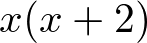Let’s focus all our attention on this for a second. The right hand side of our equation doesn’t matter right now, all we care about is the left hand side expression.

We need to multiply everything inside the brackets by everything immediately outside those brackets (that means anything that doesn’t have a sign of some sort between itself and our pair of brackets). In this case, that’s just ‘x’. Give it a go now, and you should come up with this: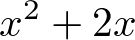Now, remember before I went on a tangent about how sofas and couches are the same? This is why: we don’t want to change anything on the right hand side of the equation right now, and for us to be able to do that, we can’t change anything on the left hand side, we can just rewrite it differently.

So to make sure we’ve only rewritten the left-hand side differently, instead of changing it, pick any number you like and substitute it into both what we started with on the left hand side, and what we ended up with on the left hand side.

If we’ve really just rewritten the same thing in different words, you should find that you get the same number out.

Our whole equation now looks like this: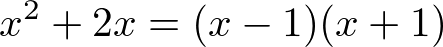Which is exactly the same as this, just written differently:### The Right Hand Side

So far, so good. Let’s look at the right hand side. We’ll follow the same steps, but this time we have two brackets to multiply out. This means we’ll use a technique known as cross-multiplication.

This means that we need to multiply everything in one bracket by everything in the other bracket. This can get a bit confusing to write down, so here’s a diagram I made earlier:We’re going to take everything in the first bracket, and multiply it by everything in the second bracket. Because multiplication is associative (this means that it works in any direction, unlike division, for example). If you’re unsure on this, it’s probably best to read up about order of operations.

The red lines in the diagram above show when we multiply the ‘x’ in the first bracket, and the blue lines show when we multiply the ‘-1’ in the first bracket. As you can see, all terms in both brackets are multiplied, so we know that we’re going to expand the bracket correctly.

This leaves us with the following expressions, starting with the ‘x’ in the first bracket: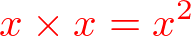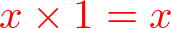And now multiplying ‘-1’ in the first bracket: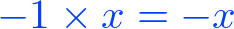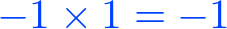So those are all the terms of the brackets on the right hand side, once they’ve been expanded. Now we can combine those into one expression, which represents the right hand side of the original equation: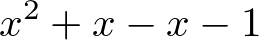### Putting It All Together

Fear not, we’re nearly there! Let’s put the left and right hand sides of our equation back together, now that we have expanded the brackets: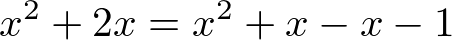Once again, that’s exactly the same as what we started with, we’ve just removed all the brackets:So, we’re free of any brackets and fractions, multiplication and division signs, and we’re more or less at a point where we can solve it. Notice how so far, all we’ve done is repeat the key steps we outlined at the start of this section?

Looking at the right hand side of the equation, adding ‘x’ and then immediately subtracting ‘x’ cancel each other out, just like multiplying and dividing do. Let’s simplify it: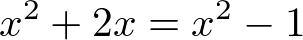Now, thinking back to the key steps we outlined for solving all equations, we need to try and isolate ‘x’ on one side of the equation. Before we start:

x2 and x are not the same thing!

You cannot combine terms with a power into terms with a different (or no) power. Let’s try and isolate ‘x’ by putting anything with an ‘x’ in it on the left hand side, and leaving anything without an ‘x’ on the right hand side.

Remember, any operation I’m doing to one side of the equation, I have to do to the other: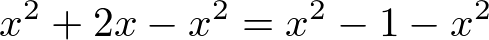Here, I’m taking x2 from both sides of the equation. This is because I’m trying to remove x2 from the right hand side by subtracting it, and since I’m subtracting x2 from one side, I have to do the same to the other.

Let’s tidy both sides of our equation up again. We have some terms that will cancel each other out on both sides. Which ones do you think they are?

Here’s how it should look after tidying: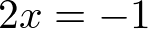Good news! We’ve isolated ‘x’ onto one side of the equation, and helpfully, the x2‘s have cancelled themselves out. That won’t always happen, sometimes you’ll need to factorise.

So our final step: ‘x’ is on one side of the equation now, but that pesky two is still there! How do we get rid of it? By dividing the left hand side by two! Hopefully you know by now that means dividing the right hand side by two as well, leaving us with our answer…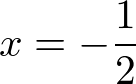## Graphing Polynomial Equations

Did you know: with the right combination of equations, you can draw any shape you like on a graph, just by using algebraic expressions? Triangles, quadrilaterals, any polygons you like!

All right with us so far? If not, consider getting a maths tutor to help walk you through it again – some people need to hear things rather than read them to learn effectively.

So, now we’re familiar with equations, here’s a crash course in graphing a polynomial equation. First, a refresher on polynomial equations:

A polynomial equation is any equation that contains at least one term with an exponent that is two or higher.

This includes, but isn’t limited to, the following types of equation:

• Asymptotic equations
• Equations of trigonometric functions
• Logarithmic equations

So, how do we graph an equation like this? We need to find the extreme points (ie: the minimum and maximum points) of the curve, and then sketch the curve.

To help us sketch the curve, we’re going to create a table of values. To do this, we’ll substitute values of ‘x’ into an equation, giving us their ‘y’ value. Once we’ve done this enough times, we’ll be able to trace out the rough shape of the curve, being sure to hit those min and max values.

Here’s all of the steps, in order, where your original equation is labelled f(x), and the derived function is f'(x):

1. Differentiate f(x)
2. Factorise f'(x) to find the minimum and maximum values of the curve
3. Substitute values into f(x) to find points on the curve
4. Mark these points on a graph
5. Sketch the shape of the curve, being sure to hit the minimum and maximum points

As always, attention to detail is key here. Make sure you plot the points correctly on the graph, and that your curve passes through the minimum and maximum points. You’ll be marked on the accuracy of your sketch, so avoid making silly errors!

Why not consider maths tutors to help you with your revision for A-level and maths revision GCSE.

Share(No ratings so far)Loading...